Latest Banking jobs   »

# Quantitative Aptitude Quiz For Bank Foundation 2023 -17th May

Directions (1-5): In the given questions, two quantities are given, one as Quantity I and another as Quantity II. You have to determine relationship between two quantities and choose the appropriate option.
(a) Quantity I > Quantity II
(b) Quantity I < Quantity II
(c) Quantity I ≥ Quantity II
(d) Quantity I ≤ Quantity II
(e) Quantity I = Quantity II or no relation

Q1. 7x men can complete a piece of work in x/4 days while 3y men can complete the same work in 3y/7 days.
Quantity I: Value of 7/6 x
Quantity II: Value of y + 5

Q2. Quantity I : Sum of first 50 odd natural numbers.
Quantity II : sum of first 50 even natural numbers.

Q3. Quantity I: A can do a piece of work in 4 days and B can do the same piece of work in 6 days. C is 20 % more efficient than B, no. of days to complete the work when all work together.
Quantity II: P can do a piece of work in 15 days while Q can do the same piece of work in 22 days, working together no. of days required by P and Q to complete the work.

Q4. Quantity I (in km/hr.): A train crosses a pole in 8 seconds and a 200m long platform in 28 seconds. What is speed of the train? (km/hr)
Quantity II (in days): P can complete a work in 45 days and P and Q together can complete the same work in 18 days. In how many days Q alone will do the same work?

Q5. Quantity I (in cm): area of rectangle is 270cm² and difference between length and breadth is 3cm. Find the sum of length and breadth?
Quantity II (y): y²-31y – 66 = 0

Directions (6-10):- Each of the following questions is provided with 2 statements i.e. Statement I & Statement II. You have to study them and find which statement (s) is/are necessary to answer the question as per the instruction set given below.
(a) Either Statement I or Statement II is sufficient to answer
(b) Only Statement I is necessary
(c) Both Statement I & Statement II are necessary to answer
(d) Neither Statement I nor Statement II is sufficient to answer
(e) Only Statement II is necessary

Q6. What is speed of car?
Statement I: The car can cover a certain distance in 4 hours.
Statement II: The car can cover same distance in 3 hour if speed increases by 5 kmph.

Q7. What is the cost price of mobile?
Statement I: the mobile is sold at a profit of 20%.
Statement II: the product is marked up at Rs 5000.

Q8. At what time the train reach station B?
Statement I: The train left station A at 8:00 AM and distance between A and B is 400 kms.
Statement II: the train can cover a distance of 100 kms in 75 minutes.

Q9. What is time taken by boat to cover 40 km downstream?
Statement I: the speed of boat in still water and speed of stream is in ratio 5 : 1. It can cover a distance of 20 km upstream in 2.5 hour.
Statement II: the boat takes 4 hour to cover a certain distance in downstream and 6 hours to return.

Q10. How many bags are there in the box?
Statement I: there are 10 pink bags in the box.
Statement II: The probability of drawing 2 red bags from 5 red bags is 2/9.

Solutions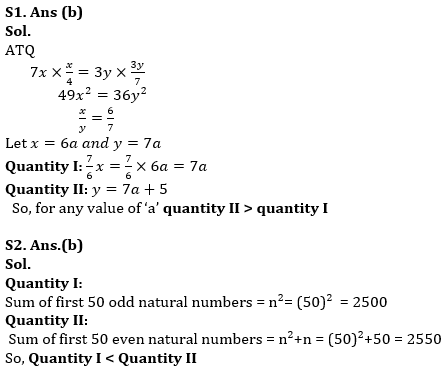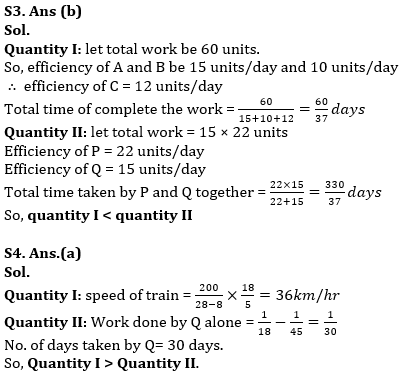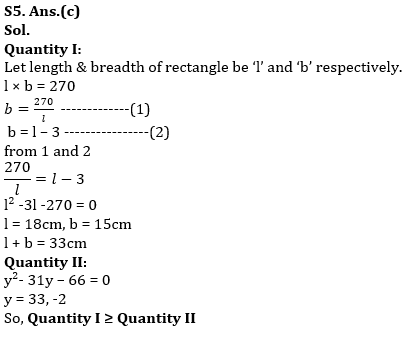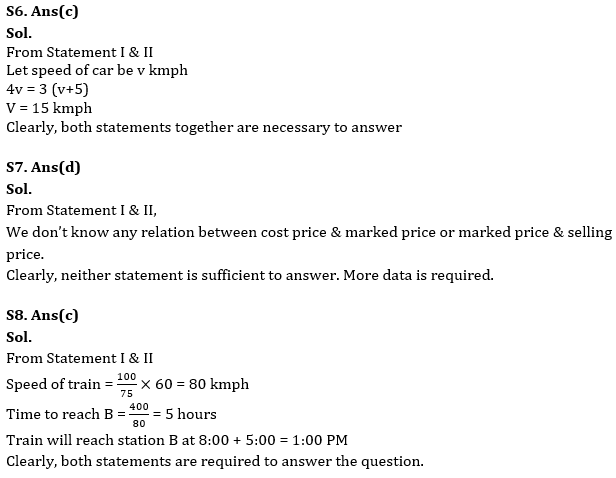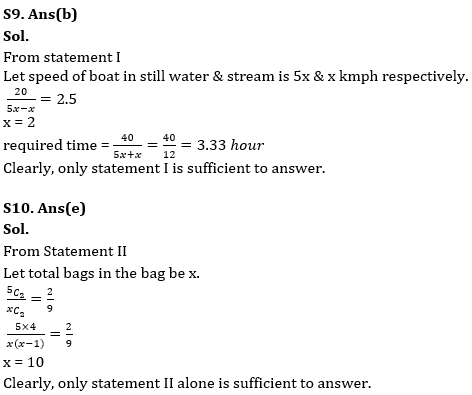## FAQs

### What is the selection process of the Bank Clerk?

The selection process of the Bank Clerk is Prelims & Mains.

#### Congratulations!Union Budget 2023-24: Free PDF